﻿

###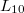$L_{10}$-free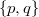$\{p,q\}$-groups

#### Abstract

If$L$ is a lattice, a group is called$L$-free if its subgroup lattice has no sublattice isomorphic to$L$. It is easy to see that$L_{10}$, the subgroup lattice of the dihedral group of order 8, is the largest lattice$L$ such that every finite$L$-free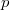$p$-group is modular. In this paper we continue the study of$L_{10}$-free groups. We determine all finite$L_{10}$-free$\{p,q\}$-groups for primes$p$ and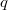$q$, except those of order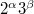$2^{\alpha}3^{\beta}$ with normal Sylow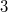$3$-subgroup

DOI Code: 10.1285/i15900932v30n1supplp55

Keywords: subgroup lattice; sublattice; finite group; modular Sylow subgroup

Full Text: PDFThis work is licensed under a Creative Commons Attribuzione - Non commerciale - Non opere derivate 3.0 Italia License.